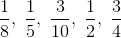# High School Math : High School Math

## Example Questions

### Example Question #71 : High School Math

Combine like terms for the simplest form: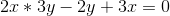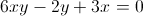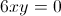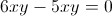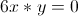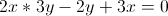Explanation:

First multiply according to order of operations to get 6xy, and then see if there are like terms to be combined. In this case, there are not so the simplest form is### Example Question #72 : High School Math

All of the following numbers are prime EXCEPT:

421

401

427

349

347

427

Explanation:

A number is prime if it is divisible by only itself and one. Thus, if a number is divisible by anything else, it can't be prime. Of the answer choices, only 427 isn't prime, because it is divisible by 7.

To figure out which number is prime, one strategy you could employ is using your calculator and dividing each choice by 3, 7, 9, 11, and 13. Because all of the answer choices are odd, we know none of them will be divisible by 2, 4, 6, 8, or 10. Also, none of them have a 0 or 5 in the ones place, so they can't be divisible by 5. Thus, the best numbers to try would be 3, 7, 9, 11, and 13. When you divide 427 by 7, you will get a whole number. For all the other answer choices, when you divide by 3, 7, 9, 11, and 13, you will never get a whole number.

### Example Question #73 : High School Math

Simplify the following expression.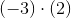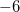Explanation:

Recall that the product of a negative number and a positive number is a negative number. Thus, we know that our answer will be a negative number. We then consider the product of the numbers, ignoring the sign. We know that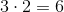. Then, we have that our final answer is### Example Question #74 : High School Math

Simplify: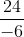Explanation:

We know that dividing a positive integer and a negative integer will give us a negative integer. We thus consider the numbers themselves, without the sign.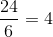Add a negative sign to find our answer is.

### Example Question #75 : High School Math

Find the product ofand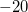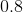Explanation:

The word product indicates multiplication. Thus, we remember that when we multiply a positive number by a negative number we get a negative number.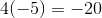### Example Question #76 : High School Math

Which of the following numbers is the greatest?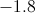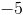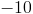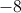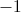Explanation:

When comparing negative numbers, it is important to remember that the numbers with the larger absolute value (greatest numerical term) are actually more negative. Though it seems that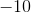would be the largest number in this case, it is actually the smallest, as it is the farthest down the number line in the negative direction. The greatest number is the one with the smallest absolute value, which is.

Remember, also, that zero is greater than any negative number!

### Example Question #77 : High School Math

Given thatis an odd integer, which of the following must produce an even integer?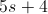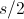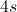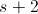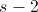Explanation:

An easy way to solve this problem is to defineas 1, which is an odd integer. Plugging in 1 into each answer yields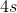as the correct answer, since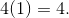4 is an even integer, which is what we are looking for.

### Example Question #78 : High School Math

Which number is greater?orIt cannot be determined from the information provided.

They are equivalent.Explanation:

When comparing negative numbers, recall the number line. Numbers that are "more negative", or negative with a large absolute value, are really very small. Thus, comparing negative numbers can sometimes seem counterintuitive. In this case, since we are comparing two negative numbers, the number with the larger absolute value is actually the smaller number. Therefore, the greater number is.

### Example Question #79 : High School Math

Which ofandis larger?NeitherExplanation:

Recall that when comparing negative numbers, those numbers that are "more negative" are actually further to the left on the number line and thus are smaller. Therefore, even though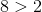, we have that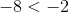Thereforeis larger.

### Example Question #80 : High School Math

Place in order from smallest to largest: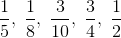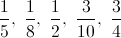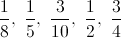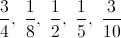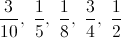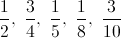Explanation:

Find the least common denominator (LCD) and convert all fractions to the LCD.  Then order the numerators from the smallest to the largest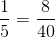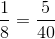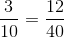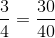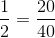So the correct order from smallest to largest is